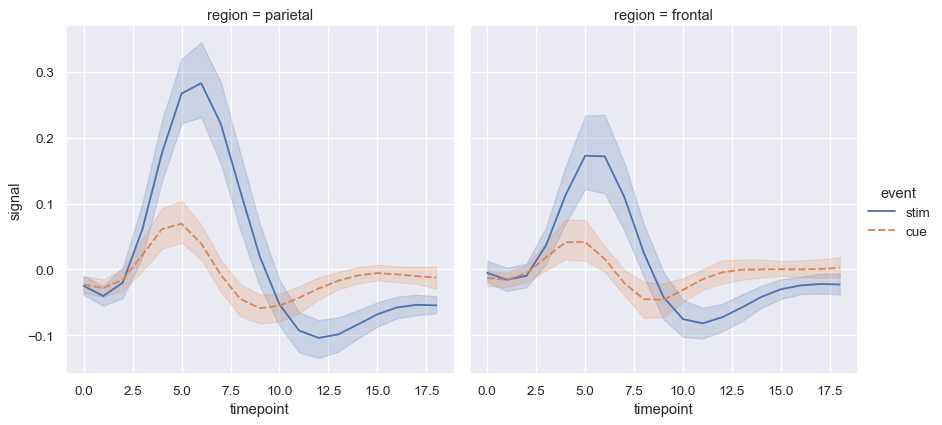# seaborn.lineplot#

seaborn.lineplot(data=None, *, x=None, y=None, hue=None, size=None, style=None, units=None, palette=None, hue_order=None, hue_norm=None, sizes=None, size_order=None, size_norm=None, dashes=True, markers=None, style_order=None, estimator='mean', errorbar=('ci', 95), n_boot=1000, seed=None, orient='x', sort=True, err_style='band', err_kws=None, legend='auto', ci='deprecated', ax=None, **kwargs)#

Draw a line plot with possibility of several semantic groupings.

The relationship between `x` and `y` can be shown for different subsets of the data using the `hue`, `size`, and `style` parameters. These parameters control what visual semantics are used to identify the different subsets. It is possible to show up to three dimensions independently by using all three semantic types, but this style of plot can be hard to interpret and is often ineffective. Using redundant semantics (i.e. both `hue` and `style` for the same variable) can be helpful for making graphics more accessible.

The default treatment of the `hue` (and to a lesser extent, `size`) semantic, if present, depends on whether the variable is inferred to represent “numeric” or “categorical” data. In particular, numeric variables are represented with a sequential colormap by default, and the legend entries show regular “ticks” with values that may or may not exist in the data. This behavior can be controlled through various parameters, as described and illustrated below.

By default, the plot aggregates over multiple `y` values at each value of `x` and shows an estimate of the central tendency and a confidence interval for that estimate.

Parameters:
data`pandas.DataFrame`, `numpy.ndarray`, mapping, or sequence

Input data structure. Either a long-form collection of vectors that can be assigned to named variables or a wide-form dataset that will be internally reshaped.

x, yvectors or keys in `data`

Variables that specify positions on the x and y axes.

huevector or key in `data`

Grouping variable that will produce lines with different colors. Can be either categorical or numeric, although color mapping will behave differently in latter case.

sizevector or key in `data`

Grouping variable that will produce lines with different widths. Can be either categorical or numeric, although size mapping will behave differently in latter case.

stylevector or key in `data`

Grouping variable that will produce lines with different dashes and/or markers. Can have a numeric dtype but will always be treated as categorical.

unitsvector or key in `data`

Grouping variable identifying sampling units. When used, a separate line will be drawn for each unit with appropriate semantics, but no legend entry will be added. Useful for showing distribution of experimental replicates when exact identities are not needed.

palettestring, list, dict, or `matplotlib.colors.Colormap`

Method for choosing the colors to use when mapping the `hue` semantic. String values are passed to `color_palette()`. List or dict values imply categorical mapping, while a colormap object implies numeric mapping.

hue_ordervector of strings

Specify the order of processing and plotting for categorical levels of the `hue` semantic.

hue_norm

Either a pair of values that set the normalization range in data units or an object that will map from data units into a [0, 1] interval. Usage implies numeric mapping.

sizeslist, dict, or tuple

An object that determines how sizes are chosen when `size` is used. List or dict arguments should provide a size for each unique data value, which forces a categorical interpretation. The argument may also be a min, max tuple.

size_orderlist

Specified order for appearance of the `size` variable levels, otherwise they are determined from the data. Not relevant when the `size` variable is numeric.

size_normtuple or Normalize object

Normalization in data units for scaling plot objects when the `size` variable is numeric.

dashesboolean, list, or dictionary

Object determining how to draw the lines for different levels of the `style` variable. Setting to `True` will use default dash codes, or you can pass a list of dash codes or a dictionary mapping levels of the `style` variable to dash codes. Setting to `False` will use solid lines for all subsets. Dashes are specified as in matplotlib: a tuple of `(segment, gap)` lengths, or an empty string to draw a solid line.

markersboolean, list, or dictionary

Object determining how to draw the markers for different levels of the `style` variable. Setting to `True` will use default markers, or you can pass a list of markers or a dictionary mapping levels of the `style` variable to markers. Setting to `False` will draw marker-less lines. Markers are specified as in matplotlib.

style_orderlist

Specified order for appearance of the `style` variable levels otherwise they are determined from the data. Not relevant when the `style` variable is numeric.

estimatorname of pandas method or callable or None

Method for aggregating across multiple observations of the `y` variable at the same `x` level. If `None`, all observations will be drawn.

errorbarstring, (string, number) tuple, or callable

Name of errorbar method (either “ci”, “pi”, “se”, or “sd”), or a tuple with a method name and a level parameter, or a function that maps from a vector to a (min, max) interval.

n_bootint

Number of bootstraps to use for computing the confidence interval.

seedint, numpy.random.Generator, or numpy.random.RandomState

Seed or random number generator for reproducible bootstrapping.

orient“x” or “y”

Dimension along which the data are sorted / aggregated. Equivalently, the “independent variable” of the resulting function.

sortboolean

If True, the data will be sorted by the x and y variables, otherwise lines will connect points in the order they appear in the dataset.

err_style“band” or “bars”

Whether to draw the confidence intervals with translucent error bands or discrete error bars.

err_kwsdict of keyword arguments

Additional parameters to control the aesthetics of the error bars. The kwargs are passed either to `matplotlib.axes.Axes.fill_between()` or `matplotlib.axes.Axes.errorbar()`, depending on `err_style`.

legend“auto”, “brief”, “full”, or False

How to draw the legend. If “brief”, numeric `hue` and `size` variables will be represented with a sample of evenly spaced values. If “full”, every group will get an entry in the legend. If “auto”, choose between brief or full representation based on number of levels. If `False`, no legend data is added and no legend is drawn.

ciint or “sd” or None

Size of the confidence interval to draw when aggregating.

Deprecated since version 0.12.0: Use the new `errorbar` parameter for more flexibility.

ax`matplotlib.axes.Axes`

Pre-existing axes for the plot. Otherwise, call `matplotlib.pyplot.gca()` internally.

kwargskey, value mappings

Other keyword arguments are passed down to `matplotlib.axes.Axes.plot()`.

Returns:
`matplotlib.axes.Axes`

The matplotlib axes containing the plot.

`scatterplot`

Plot data using points.

`pointplot`

Plot point estimates and CIs using markers and lines.

Examples

The `flights` dataset has 10 years of monthly airline passenger data:

```flights = sns.load_dataset("flights")
```
year month passengers
0 1949 Jan 112
1 1949 Feb 118
2 1949 Mar 132
3 1949 Apr 129
4 1949 May 121

To draw a line plot using long-form data, assign the `x` and `y` variables:

```may_flights = flights.query("month == 'May'")
sns.lineplot(data=may_flights, x="year", y="passengers")
```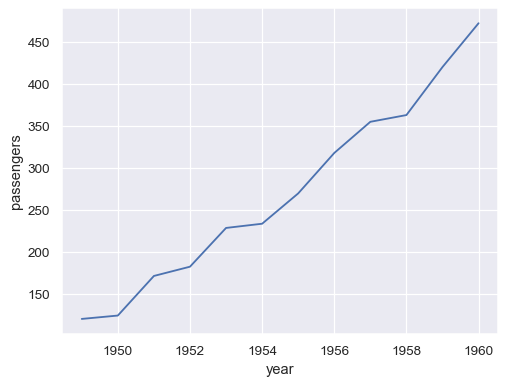Pivot the dataframe to a wide-form representation:

```flights_wide = flights.pivot("year", "month", "passengers")
```
```/var/folders/qk/cdrdfhfn5g554pnb30pp4ylr0000gn/T/ipykernel_77739/1230890992.py:1: FutureWarning: In a future version of pandas all arguments of DataFrame.pivot will be keyword-only.
flights_wide = flights.pivot("year", "month", "passengers")
```
month Jan Feb Mar Apr May Jun Jul Aug Sep Oct Nov Dec
year
1949 112 118 132 129 121 135 148 148 136 119 104 118
1950 115 126 141 135 125 149 170 170 158 133 114 140
1951 145 150 178 163 172 178 199 199 184 162 146 166
1952 171 180 193 181 183 218 230 242 209 191 172 194
1953 196 196 236 235 229 243 264 272 237 211 180 201

To plot a single vector, pass it to `data`. If the vector is a `pandas.Series`, it will be plotted against its index:

```sns.lineplot(data=flights_wide["May"])
```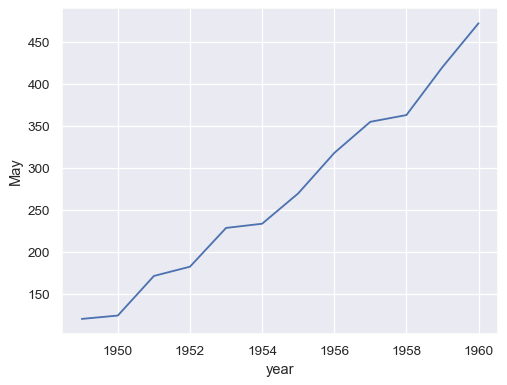Passing the entire wide-form dataset to `data` plots a separate line for each column:

```sns.lineplot(data=flights_wide)
```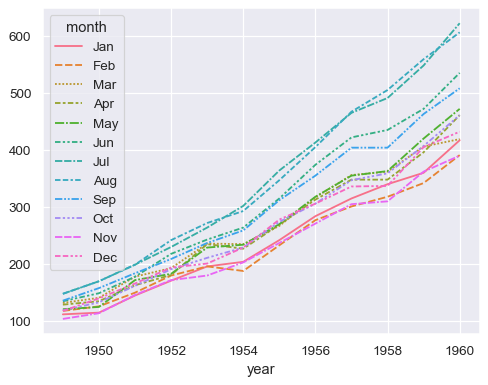Passing the entire dataset in long-form mode will aggregate over repeated values (each year) to show the mean and 95% confidence interval:

```sns.lineplot(data=flights, x="year", y="passengers")
```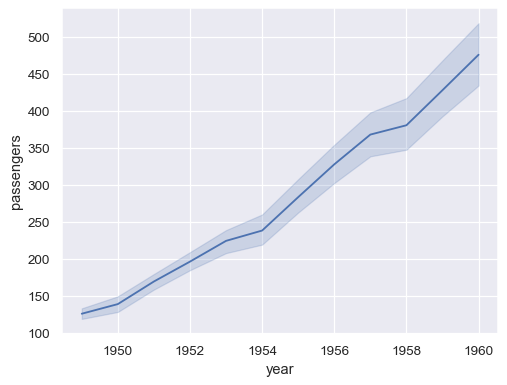Assign a grouping semantic (`hue`, `size`, or `style`) to plot separate lines

```sns.lineplot(data=flights, x="year", y="passengers", hue="month")
```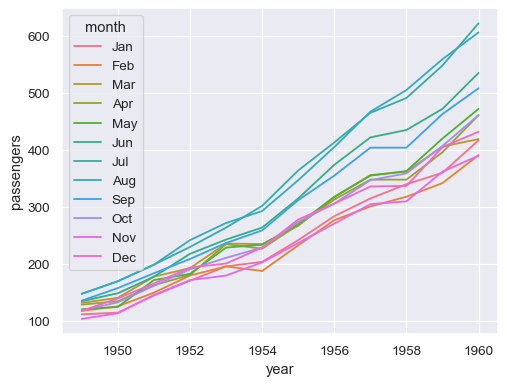The same column can be assigned to multiple semantic variables, which can increase the accessibility of the plot:

```sns.lineplot(data=flights, x="year", y="passengers", hue="month", style="month")
```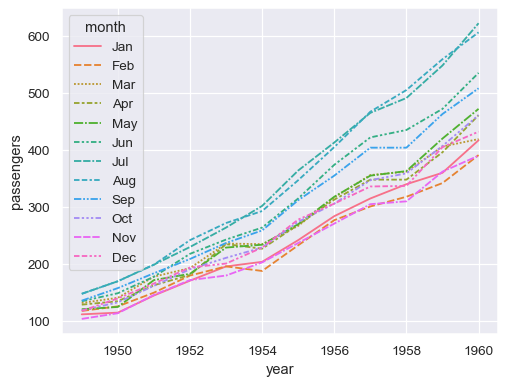Use the `orient` parameter to aggregate and sort along the vertical dimension of the plot:

```sns.lineplot(data=flights, x="passengers", y="year", orient="y")
```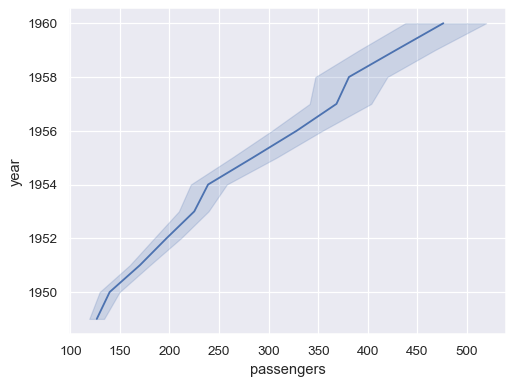Each semantic variable can also represent a different column. For that, we’ll need a more complex dataset:

```fmri = sns.load_dataset("fmri")
```
subject timepoint event region signal
0 s13 18 stim parietal -0.017552
1 s5 14 stim parietal -0.080883
2 s12 18 stim parietal -0.081033
3 s11 18 stim parietal -0.046134
4 s10 18 stim parietal -0.037970

Repeated observations are aggregated even when semantic grouping is used:

```sns.lineplot(data=fmri, x="timepoint", y="signal", hue="event")
```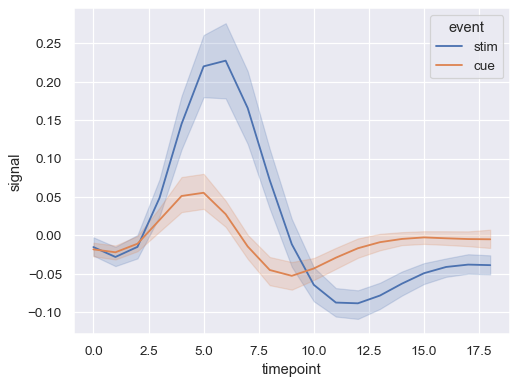Assign both `hue` and `style` to represent two different grouping variables:

```sns.lineplot(data=fmri, x="timepoint", y="signal", hue="region", style="event")
```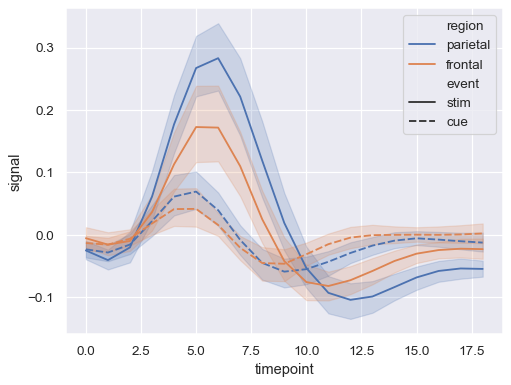When assigning a `style` variable, markers can be used instead of (or along with) dashes to distinguish the groups:

```sns.lineplot(
data=fmri,
x="timepoint", y="signal", hue="event", style="event",
markers=True, dashes=False
)
```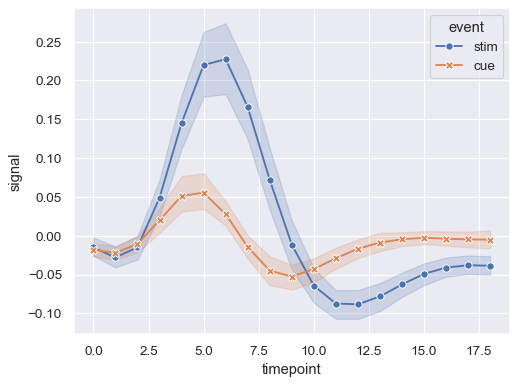Show error bars instead of error bands and extend them to two standard error widths:

```sns.lineplot(
data=fmri, x="timepoint", y="signal", hue="event", err_style="bars", errorbar=("se", 2),
)
```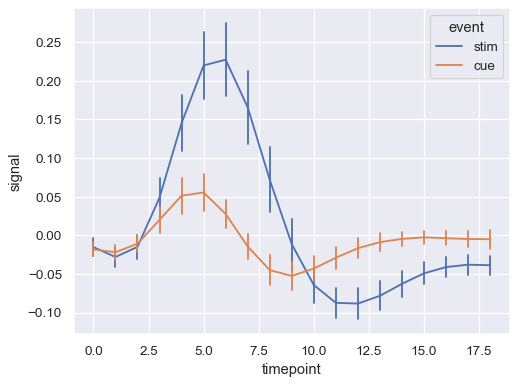Assigning the `units` variable will plot multiple lines without applying a semantic mapping:

```sns.lineplot(
data=fmri.query("region == 'frontal'"),
x="timepoint", y="signal", hue="event", units="subject",
estimator=None, lw=1,
)
```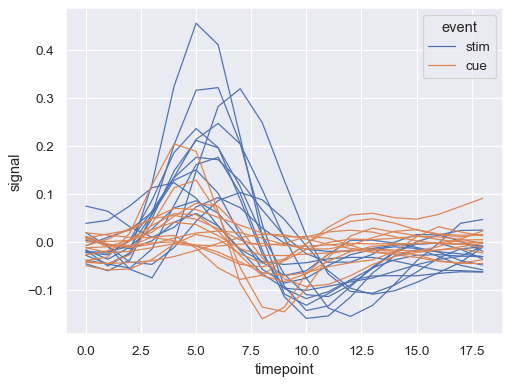Load another dataset with a numeric grouping variable:

```dots = sns.load_dataset("dots").query("align == 'dots'")
```
align choice time coherence firing_rate
0 dots T1 -80 0.0 33.189967
1 dots T1 -80 3.2 31.691726
2 dots T1 -80 6.4 34.279840
3 dots T1 -80 12.8 32.631874
4 dots T1 -80 25.6 35.060487

Assigning a numeric variable to `hue` maps it differently, using a different default palette and a quantitative color mapping:

```sns.lineplot(
data=dots, x="time", y="firing_rate", hue="coherence", style="choice",
)
```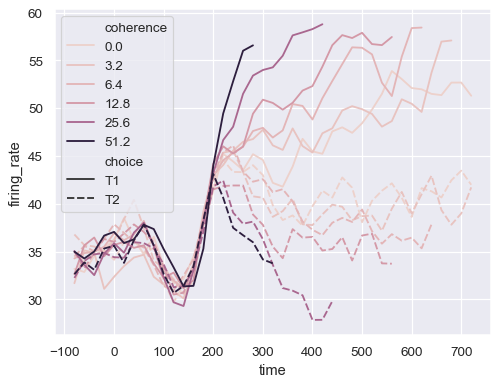Control the color mapping by setting the `palette` and passing a `matplotlib.colors.Normalize` object:

```sns.lineplot(
data=dots.query("coherence > 0"),
x="time", y="firing_rate", hue="coherence", style="choice",
palette="flare", hue_norm=mpl.colors.LogNorm(),
)
```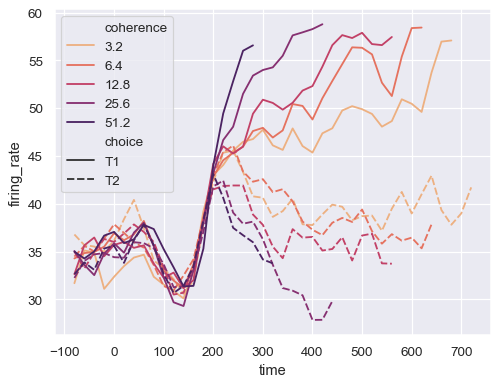Or pass specific colors, either as a Python list or dictionary:

```palette = sns.color_palette("mako_r", 6)
sns.lineplot(
data=dots, x="time", y="firing_rate",
hue="coherence", style="choice",
palette=palette
)
```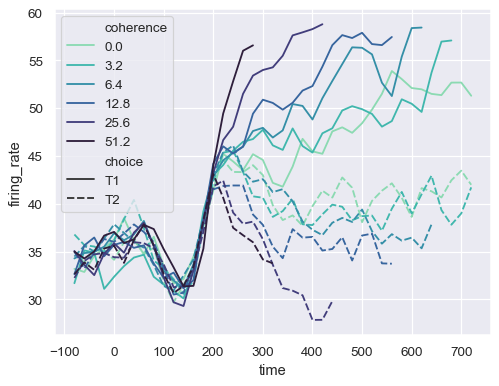Assign the `size` semantic to map the width of the lines with a numeric variable:

```sns.lineplot(
data=dots, x="time", y="firing_rate",
size="coherence", hue="choice",
legend="full"
)
```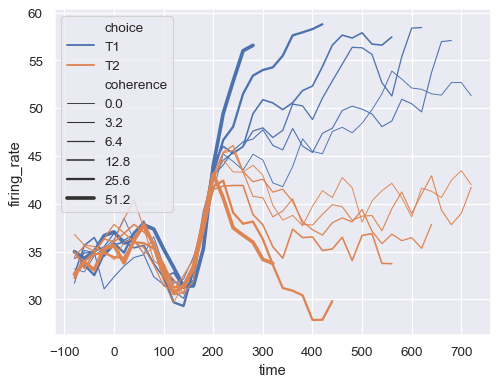Pass a a tuple, `sizes=(smallest, largest)`, to control the range of linewidths used to map the `size` semantic:

```sns.lineplot(
data=dots, x="time", y="firing_rate",
size="coherence", hue="choice",
sizes=(.25, 2.5)
)
```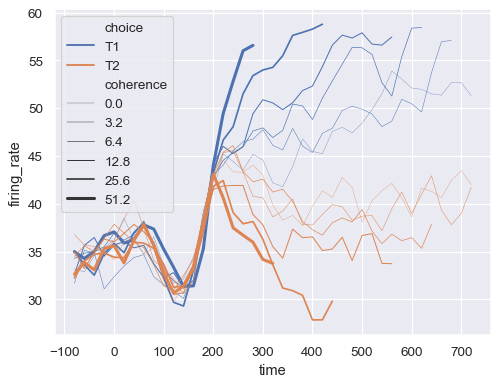By default, the observations are sorted by `x`. Disable this to plot a line with the order that observations appear in the dataset:

```x, y = np.random.normal(size=(2, 5000)).cumsum(axis=1)
sns.lineplot(x=x, y=y, sort=False, lw=1)
```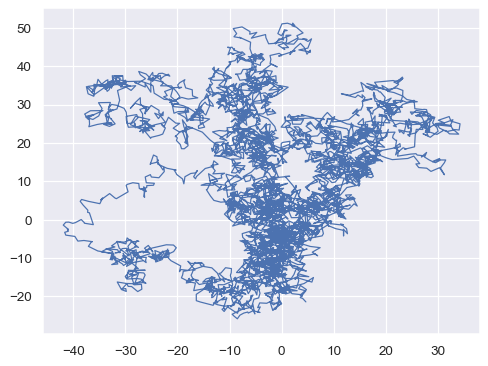Use `relplot()` to combine `lineplot()` and `FacetGrid`. This allows grouping within additional categorical variables. Using `relplot()` is safer than using `FacetGrid` directly, as it ensures synchronization of the semantic mappings across facets:

```sns.relplot(
data=fmri, x="timepoint", y="signal",
col="region", hue="event", style="event",
kind="line"
)
```